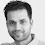## Saturday, August 08, 2009

### Negative-type distances and kernels

This is a story of two constructions that actually end up being essentially the same thing, as I recently discovered.

1. Kernels

The story of kernels in machine learning goes somewhat like this:

Take a positive definite function $$K(x,y)$$ that captures some notion of similarity between objects (a standard example of such a kernel is the Gaussian $$K(x,y) = \exp(-\|x - y\|^2)$$). A positive definite function, btw, is like the generalization of a p.d matrix: the integral $$\int f(x)f(y)k(x,y)dx dy \ge 0$$.

You can then construct a Hilbert space that captures the structure of the kernel. Specifically, for a fixed set of points S, construct a vector space from the basis $$\{k_x | x \in S\}$$, where $$k_x(y) = K(x,y)$$, and then define an inner product of two vectors in this space in the usual way: If $$v_a = \sum a_x k_x$$ and $$v_b = \sum b_x k_x$$, then $$v_a \cdot v_b = \sum a_x b_y K(x,y)$$.

You get nice properties from this construction: the so-called reproducing property is one of them, which tells you that $$K(x,y) = k_x \cdot k_y$$. In other words, we can capture the similarity function K(x,y) by a "standard" inner product in a Hilbert space.

What's even neater is that by invoking Mercer's theorem, you can construct an orthogonal basis, and make sure that the Hilbert space is actually a Euclidean space. The squared Euclidean distance in this space can be written in kernel form, as
$d_K(x,y) = K(x,x) + K(y,y) - 2K(x,y)$
which is what you'd expect when treating K(.) as an inner product.

2. Negative-type distance.
The second story comes from Deza and Laurent. When can a distance function be embedded in Euclidean space ? It turns out that it's more convenient to talk about the square of the distance function, which we'll call D.

There's an elegant characterization of when D can be embedded isometrically into $$\ell^2_2$$: this can be done if and only if D satisfies the negative-type inequality:
$\sum b_i b_j D(x_i, x_j) \le 0, \sum b_i = 0$
for all possible assignments to $$b_i$$.

The proof works via a construction called a covariance mapping that takes $D$ to the function $$k_D$$ defined as:
$k_D(x,y) = (1/2)(d(x, x_0) + d(y, x_0) - d(x,y))$
which differential geometry folks will recognize as the Gromov product.

The proof completes by showing that the negative-type condition on D implies positive definiteness of $$k_D$$, and this in turn means that $$k_D$$ can be expressed as an R-covariance:
$k_D(x,y) = \int f_x(\omega)f_y(\omega) d\mu(\omega)$
for some measure space $\mu$.

Note that the RHS of the equation is an infinite-dimensional inner product.

3. Where the two stories come together
The mapping that takes a kernel to a distance is the inverse of the covariance mapping used to map a distance to a metric. In other words, if we take a kernel K, compute $$d_K$$, and then use the distance to kernel mapping to compute $$k_{d_K}$$, we get back K. Further, since we can show that the negative-type condition on D implies a positive-definite condition on $$k_D$$, we can start off with either "D satisfies negative-type inequalities" or "K is a positive definite kernel" and yield the same conclusion on $$\ell_2^2$$ embeddability.

What's interesting is that the two ways of thinking about this don't seem to have been connected together explicitly before.

p.s This is not a fully rigorous correspondence except possibly in the finite dimensional case, but I find that it's often convenient to replace the negative-type argument with a kernel positive-definiteness argument in my head.

1.Thanks; that's nice!

2.Just a little comment to say that I really like your Blog!! It's always a pleasure to read your posts and thanks for that!!

3.This correspondence is known at least since Schoenberg (1938), "Metric Spaces and Positive Definite Functions". It is presented in detail in the book Harmonic Analysis on Semigroups, by Berg, Christensen, and Ressel (1984), where the "squared distances" are called "negative definite kernels." This is also well known in the literature on kernel methods, e.g. Schoelkopf and Smola's Learning With Kernels (2001), where the negative of these squared distances appear under the name "conditionally positive definite kernels."

4.Indeed. maybe I should have made it clear. the correspondence is well known: it's just that I hadn't put it together till now (and reading Deza and Laurent, it's not explicit unless you're already familiar with kernels)

5.I knew that there was a connection from kernels to Euclidean distances but didn't know that it was other way around too.. Good post. Thanks.